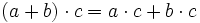# Jordan ring

## Definition

### Symbol-free definition

A Jordan ring is a non-associative ring (i.e., a not necessarily associative ring) whose multiplication gives a Jordan magma.

### Definition with symbols

A Jordan ring is a set$A$ equipped with binary operations$+$ and$\cdot$, a constant$0$, and a unary operation$-$, such that:

1.$(A,+,0,-)$ is an abelian group.
2. Distributivity laws: For all$a,b,c \in A$:
•$\! a \cdot (b + c) = a \cdot b + a \cdot c$
•$\! (a + b) \cdot c = a \cdot c + b \cdot c$.
3. Commutativity of$\cdot$: For all$a,b \in A$,$a \cdot b = b \cdot a$.
4. The Jordan identity: For all$a,b \in A$, we have:$\! (a \cdot b) \cdot (a \cdot a) = a \cdot (b \cdot (a \cdot a))$.